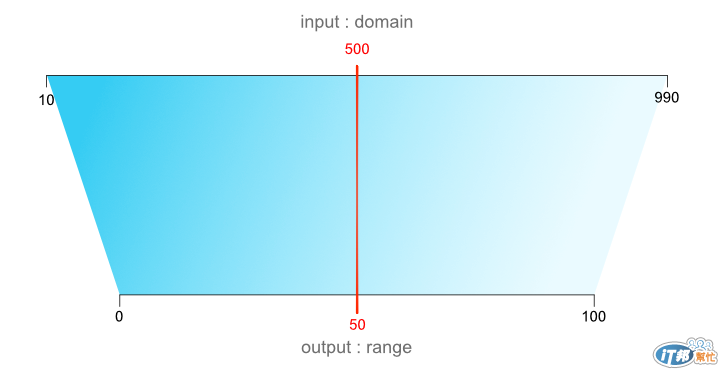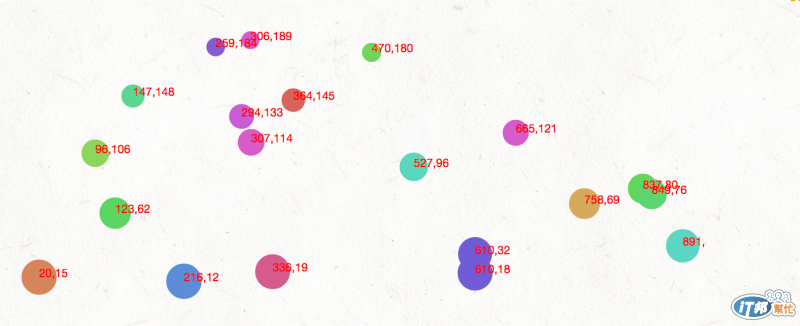#DAY 10
3

## 尺度``````var d3Scale = d3.scale.linear() //產生一個線性尺度
.domain([10, 999]) //傳入的值
.range([0 , 100]) //輸出的範圍

console.log("輸出的值 = " + d3Scale(500))
``````

## Demo``````var w = 600,h = 250,padding = 20;
//定義SVG的大小

var dataset = [];
for (var i=0; i < 20; i++){
var Num1 = 5 + Math.floor(Math.random() * 900);
var Num2 = 5 + Math.floor(Math.random() * 220);
dataset.push([Num1, Num2]);
};
console.log(dataset);
//和先前一樣，產生一組隨機的資料

var Xmax = d3.max(dataset, function(d){return d}),
Xmin = d3.min(dataset, function(d){return d}),
Ymax = d3.max(dataset, function(d){return d}),
Ymin = d3.min(dataset, function(d){return d})
//這個函示可以取得資料的最大值、最小值

var xScale = d3.scale.linear() //產生一個屬於X軸的線性尺度
.domain([Xmin, Xmax]) //傳入的值是原始資料的最小及最大值

var yScale = d3.scale.linear()
.domain([Ymin, Ymax])
//類似X軸的尺度

var svg = d3.select('.demo').append('svg').attr({'width': w,'height': h})
//接下來開始產生SVG

svg.selectAll('circle').data(dataset).enter() //和先前一樣，選取'circle'並把資料加入
.append('circle') // 增加圓到SVG內
.attr({	//加入屬性到圓
'cx': function(d){return xScale(d)}, //利用尺度算出X的位置
'cy': function(d){return yScale(d)}, //同理算出Y
'r': function(d){return Math.sqrt(h - d)}, //圓的大小是高 - Y值的平方
'fill': function(d){return d3.hsl(d % 360, .6, .6 );}
//介紹一個顏色的function hsl，可以將顏色算出後轉成色碼
//格式 (360色相, 1彩度, 1明度)
});

svg.selectAll('text').data(dataset).enter() //補上資料數值
.append('text')
.text(function(d){ return d+ ',' + d}) //將值寫到SVG上
.attr({
'x': function(d){return xScale(d)}, //和上面相同，算出X、Y的位置
'y': function(d){return yScale(d)},
'fill': 'red', //文字填滿為紅色
'font-size': '10px' //Fill、font-size也可以用CSS寫喔～
});
``````

SVG 與 D3.js30